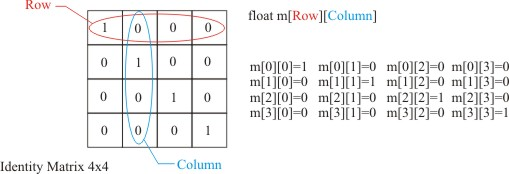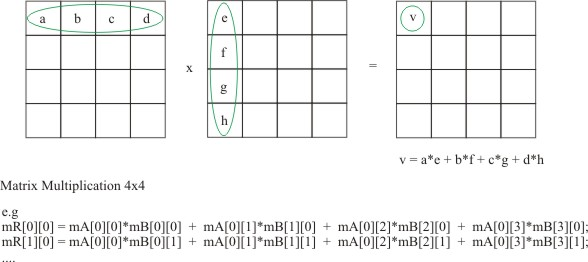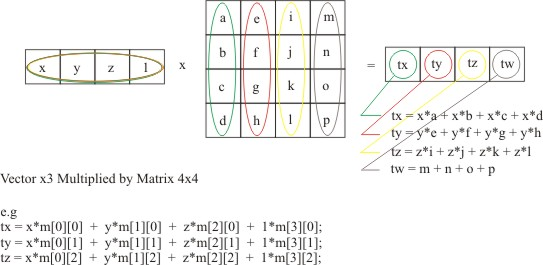Sunday March 26, 2023
 home | about | contact | DonationsSlice of Java. Its simple and its powerful...

Better Camera - UVN! (with a cup of Matrix on the side)

by Ben Kenwright

Well this is the most popular method for camera's....and you'll see it used in things like DirectX and OpenGL.  At first it will look a bit crazy the code...and of course we'll have to use some matrix math here so its easier to work with!  But it was only a matter of time before matrix's popped up.  It allows us to combine our maths operations into a single matrix and then we can apply that single matrix to all our vertices :)  That's where the real power of matrices come from...the ability to combine them, so we can do all our tricky maths in one fast go.  We will use a 4x4 matrix class, and keep things simple.  Sometimes people use 4x3 or 3x3 for space and speed etc...but the 4x4 I think will be better.  I dont' want to go into all the theory of homogenious w values or Vector3 or Vector4.  What we want is a working model :)....code that works...and does what we want...and the Matrix4 will meet all our needs.

So this is what a basic matrix is, under all those built in functions and wrappers:

 Basic Matrix4: class Matrix4 {     public float[][] m; };

Thats all there is to it....of course this is java, so we of course need to allocate room for the array...but I just wanted to show you that our 4x4 Matrix, is just a bunch of floats.  How we use these floats is the next part.

As we are working with Java, its object orientated, so its only obvious that we make the Matrix4 class contain its own useful little methods, like multiplying matrix's together...and building a rotation matrix.

Just to sort this out now - get this clear in your heads!  Columns and Rows!  You have to make sure you know which are which, and stick with that system.  As I've found that opengl uses a different system to directx...and you can on occasion be looking around on the net or in your 3d maths books on how to do things, and get mixed up with which is which.  So I would recommend doing this a few times on paper and do a few simple demos with basic matrices.

We'll have to review Matrix theory!  So hold on...this is where it gets scary....so drink lots of coffee and try to remember - its only an array of numbers....nothing more.  First we give you an identity matrix:

 Identity Matrix SetupAs any matrix multiplied by an identity matrix, we end up with what we started with.  Usually, when we start with a new Matrix, we set it to an identity matrix first, then convert or modify it to our needs.

So now that we have a matrix...lets say, two identity matrix's...how do we combine them?...well its simple:

 Matrix Multiplication BasicsOf course, you have to really really think about matrix multiplication to see how you would apply it to code.  Basically we can do it with a few for loops, making sure we keep track of columns and rows...which I've done in our Matrix4 class - adding a simple multiply member function.  Of course you could optimise it later on, as it is just a combination of Dot Products if you think about it long enough :)

 Matrix Code: /*********************************************************************************************/ /*                                                                                           */ /*  class Matrix4                                                                            */ /*  You can't do much in 3D these days without using matrix's...it basically makes it easier */ /*  to work with data, and to combine operations into a single one :)                        */ /*                                                                                           */ /*********************************************************************************************/   class Matrix4 {     public float[][] m;         // Constructors     public Matrix4()     {        m = new float;     }// End Matrix4() default constructor                 public Matrix4(float m00, float m01, float m02, float m03,                    float m10, float m11, float m12, float m13,                    float m20, float m21, float m22, float m23,                    float m30, float m31, float m32, float m33)     {        m= new float;        m=m00;  m=m01;  m=m02;  m=m03;        m=m10;  m=m11;  m=m12;  m=m13;        m=m20;  m=m21;  m=m22;  m=m23;        m=m30;  m=m31;  m=m32;  m=m33;     }// End Matrix4(..)         static public Matrix4 multiply(Matrix4 a, Matrix4 b)     {        Matrix4 result = new Matrix4();               for(int col=0; col<4; col++)        {           for(int row=0; row<4; row++)           {              result.m[row][col] = 0;              for(int i=0; i<4; i++)              {                 result.m[row][col] += a.m[row][i] * b.m[i][col];              }// End for loop i           }// End for loop row        }// End for loop col               return result;            }// End multiply(..)      // Matrix Multiplication      //      //  a b c d        e - - -      //  - - - -        f - - -      //  - - - -   x    g - - -      //  - - - -        h - - -      //      //  m00 = a*e + b*f + c*g + d*h          ......  }// End Matrix4(..)

One further question that might be on your mind, before going onto Cameras and Matrices - is the combination of our Vector3 with a Matrix4.  Well in reality we should have a Vector4, with a homogenous w in there so that its mathematically correct.  But we can use the assumption that it is always 1, in Vector3...and we can make our functional code work and do what's its suppose to...saving us a bit of space - as we don't' really use the w - and if we do, we'll just introduce a Vector4 class :)

 Vector3 multiplied by Matrix4As we use this ability to multiply a Vector3 by a Matrix4, so we can apply our Matrices to our actual vertices data.  As we have all our vertices, stored as Vector3 values - and we build up our Matrix, which performs all our tricky rotation, transition and camera operations into a single matrix - then we apply it to all our vertices using our Vector3 mul member function :)  Sounds simple eh?... well it is :)

 Vector3 member function Matrix Multiplication static public Vector3 mul( Vector3 a, Matrix4 b )    {       Vector3 result = new Vector3();             // We assume a vector of 4 with w = 1       result.m_x = a.m_x*b.m + a.m_y*b.m + a.m_z*b.m + 1*b.m;       result.m_y = a.m_x*b.m + a.m_y*b.m + a.m_z*b.m + 1*b.m;       result.m_z = a.m_x*b.m + a.m_y*b.m + a.m_z*b.m + 1*b.m;       // w = a.m_x*b.m + a.m_y*b.m + a.m_z*b.m + 1*b.m;       return result;    }// End mul(..)       //  mul(...)    //                 a - - -    //   x y z w   *   b - - -    //                 c - - -    //                 d - - -    //    //   tx = x*a + y*b + z*c + w*d

So where where we?...hmmm... Oh yeah - onto a better camera.

 UVN Camera Model: Translation matrix =           |  1  0  0  0 |         |  0  1  0  0 |         |  0  0  1  0 |         | -x -y -z  0 |     Rotation matrix =           | Ux Vx Nx 0 |         | Uy Vy Ny 0 |         | Uz Vz Nz 0  |         | 0    0   0   1  |     CameraMatrix = Translation*Rotation

Where U is the "right" vector, V the "up" vector and N the direction you are looking.

If you think about it, we have a more realistic camera - as we now have a look at point and a right and up vector.  We can still use our previous method, of and an angle and location to build or UVN matrix model.

 Building Camera UVN Matrix: static public Matrix4 BuildCameraMatrix( Vector3 rot,                                              Vector3 pos )     {        Matrix4 matCam = new Matrix4();               /* ROTATION MATRIX */        Vector3 vUp      = new Vector3(0,1,0);        Vector3 vForward = new Vector3(0,0,1);        Vector3 vRight   = new Vector3(1,0,0);            vForward = Vector3.rotatey( vForward, rot.m_y );        vRight   = Vector3.rotatey( vRight, rot.m_y );                 //vUp = Vector3.cross( vRight, vForward );        //vUp = Vector3.normalize(vRight);                            matCam.m=vRight.m_x;          matCam.m=vRight.m_y;          matCam.m=vRight.m_z;        matCam.m= 0;                            matCam.m=vUp.m_x;          matCam.m=vUp.m_y;          matCam.m=vUp.m_z;        matCam.m= 0;                            matCam.m=vForward.m_x;          matCam.m=vForward.m_y;          matCam.m=vForward.m_z;        matCam.m= 0;                        matCam.m=0;        matCam.m=0;        matCam.m=0;        matCam.m= 1;                            /* TRANSLATION MATRIX */        Matrix4 vPos = new Matrix4();        vPos = Matrix4.identity(vPos);        vPos.m = -pos.m_x;        vPos.m = -pos.m_y;        vPos.m = -pos.m_z;                            /* Combine Rot and Trans Matrix to create the Camera Matrix */        matCam = Matrix4.multiply( vPos, matCam );                            /*         | Ux  Vx  Nx |         | Uy  Vy  Ny |         | Uz  Vz  Nz |                  Where U is the "right" vector, V the "up" vector and N the         direction you are looking.        */                            return matCam;     }// End BuildCameraMatrix(..)         //  Camera Matrix     //  | right.x    up.x     forward.x     0 |     //  | right.y    up.y     forward.y     0 |     //  | right.z    up.z     forward.z     0 |  ; (rotation4x4)     //  | 0          0            0         1 |

We can use this simple function, so pass our Eulers angles and our cameras position, and it will build us our UVN camera matrix.  I've excluded the two lines that build the vUp value - as for our demo's we only seem to move around the world in the x-z directions....so we can assume that our up vector of (0,1,0) doesn't change.  But if it did, we could use the cross product of vForward and vRight.

So for each object we have this:

Mat = matRot * matTran * matCamTran * matCamRotation

We could also do a Perspective matrix and further combine our matrix's, but I'm choosing to keep our perspective code separate at this time.

 Applet Demo - Download Applet Source Code ...etc....     Vector3 m_EyeAngle = new Vector3( 0, 0, 0 );   Vector3 m_EyePos   = new Vector3( 0, 0, -10 );   Vector3 m_BoxPos   = new Vector3( 0, 0, -1 );     public void update(Graphics g)   {       // Clear screen       offScreen.setColor(Color.white);       offScreen.fillRect(0,0,this.getSize().width,this.getSize().height);                         Dimension appletSize = this.getSize();       int width  = appletSize.width;       int height = appletSize.height;                           ResetAllCoords( m_tri, m_NumTris );             Matrix4 matWorld = new Matrix4();       matWorld = Matrix4.translate( m_BoxPos.m_x, m_BoxPos.m_y, m_BoxPos.m_z );             Matrix4 matCam = Camera.BuildCameraMatrix( m_EyeAngle,                                                  m_EyePos );             // Combine all our matrices to gether, into a single matrix...which we'll apply       // to all our vertices.       Matrix4 mat = new Matrix4();       mat = Matrix4.identity(mat);       mat = Matrix4.multiply(mat, matCam);       mat = Matrix4.multiply(mat, matWorld);                  // Lets rotate all of the triangles and translate them back into the horizon       for(int i=0; i

The 3D applet code is sure coming along - we're starting to build up a quit a little library of mini routines and principles here.  But thats all it is basically, small math stages, which allow us to take data and go to the 3rd dimension :)  You'll be rewarded in the end, when you get that doom level applet running in your browser!  Yup, your browser, isn't that cool eh?  And then if you extend these principles to DirectX and OpenGl you can really push up the Frames Per Second (FPS) and number of poly's into the hundreds of thousands or even millions :).